# Fractions

## Improper Fraction. Introducing whole fraction

#### Three types of fractions

Type1.  Proper fraction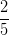, where 2 < 5 (means the numerator is smaller then the denominator)

Type2. Improper fractions, where 7 > 5(it means that the numerator is higher then the denominator)

Type 3. Mixed fractions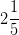, here we have whole in front of the proper fraction

#### Improper fractions

If we have a improper fraction (numerator is greater than the denominator) then we can get the whole of the fraction. To remove the whole of the fraction procedure:

•     - do the division
•     - quotient is the whole
•     - the rest of the numerator of the fraction remaining subunit (which has the same ground as the initial fraction)

If we have the fractionwith  a > b and we get: a : b = c rest r,

then:Example: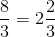because 8 : 3 = 2 rest 2.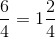#### Introducing whole in fraction

When we introducce integer in fractions we have the same denominator, but the numerator is obtained by the sum of the numerator and denominator given the product of the whole.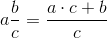Example: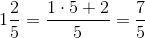.

Keywords: fractions, arithmetic, whole numbers, fractor

### Forum

Here you can discuss about mathematic, about algebra, geometry, trigonometry.

It is not mandatory to be logged in on this forum but it is nice to have an account. You can ask about mathematics just with your name and your email.

This maths forum is one of the easiest forums to use it.

>> Go to Math Forum